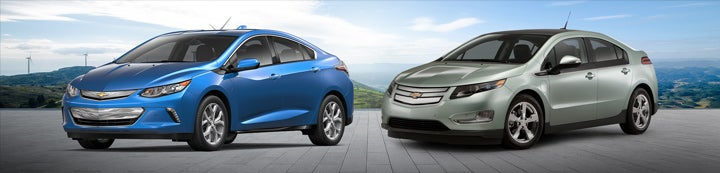1 - 7 of 7 Posts

#### kgvickers

·
##### Registered
Joined
·
157 Posts
Discussion Starter
I've often thought that a better near-term compromise for a PHEV would be a reasonable amount of battery on-board plus a small-ish, very efficient gas or diesel generator that can run anytime to charge the battery (even overnight at a hotel if needed). So I'm trying to do some real-ish math to figure out what a decent compromise would be.

I'm an EE by training and I'm embarrassed to admit I can't for the life of me recall how to set this fantasy problem up.

Pretend we have a PHEV with enough battery on board to go 300 miles at 75 mph (ignore wintertime issues). I'll assume 3 miles per kWh so then I've got 100 kWh of battery on board. I know, 100 kWh of battery is insane for what I'm talking about but let's start there for the heck of it. With a proper equation we can easily change the battery size.

To extend range I'll add only a 10 kW generator that I can run whenever I choose. Assume I've got a rather large gas tank. Assume I don't need no stinkin' battery buffer!

Also assume I've got a very big bladder.

I start driving with a full battery, full fuel tank, and empty bladder.

I'm going 75 mph so I'll be able to go for four hours before the battery is depleted. But at the start of the trip I also started the generator, so at the end of the 4 hours I've added back 40 kWh. That will move me another 120 miles. At the same 75 mph another 1.6 hours have passed and the generator has added back 16 kWh. That gives me another 48 miles of range ..... blah, blah, blah.

So how long before I'm down to attempting to run the car on nothing but the generator? I know this should be a simple linear-math equation but I'm getting nowhere trying to set this up.

#### obermd

·
##### Registered
Joined
·
3,003 Posts
You're looking at a mildly complex series of sums expansion of the basic formula. On a software development forum you'd be asked if this is a homework question.

#### Jmaj

·
##### Registered
Joined
·
1,214 Posts
At a glance I see a first order differential equation with a solution that looks like a exponential function. Maybe my physics is rusty though.

#### sparks

·
##### Registered
Joined
·
716 Posts
I think a little algebra would do the trick. Solve for T as the number of hours before the battery is exhausted.

Energy burn rate = (75 mi/hr) X (1/3 kWh/mi) = 25 kWh/hr, i.e., 25 kW.
Generator rate = 10 kWh/hr, i.e., 10 kW

100 kWh - 25T + 10T = 0
100 = 15T
T=6.66667 hrs (i.e., 6 2/3 hrs)

So the distance traveled would be 500 miles in 6 2/3 hours (at 75 MPH), at which point the battery is exhausted and you're stuck with the generator only, which would force a significant reduction in speed at that point.

#### gjm3

·
##### Registered
Joined
·
431 Posts
I’m late to the game but I agree with ‘sparks’ - simple algebra.

You can also set it up using distance.

Let the battery run out after time T hours.

In that time, the 10 kW generator will have produced 10T kWh, and so, at 3 miles/kWh, will have taken the car 30T miles. Add in the 300 miles of travel from the original battery charge to give a total of 300+30T miles.

But the car is traveling at 75 mph, so in time T will have traveled 75T miles.

Now it’s simple algebra:

75T=300+30T

So 45T=300 or T= 6.66667 hours, as ‘sparks’ said.

#### wordptom

·
##### Registered
Joined
·
1,559 Posts
Seems to me there would be charging losses associated with the recharging circuits.

When driving a Volt in CS Mode, generally speaking, the car’s motor is being fueled via generator output, not by the battery, and electricity is drawn from the battery (and then replaced by recharging from the generator output) only when the motor’s power request is greater than the generator can supply. (I ignore the finer points of how the computer uses the battery buffer, regen use, etc., to select the electricity source when in CS Mode.)

If the motor is not, in effect, plugged into and drawing that 10 kW from the generator output (supplemented when needed by battery power), then the 10 kW of generator output is flowing through the recharging circuits to recharge the battery. This will create something less than 10 kW in usable battery power. The "net" battery drain will be >15 kW at 75 mph (=25 kW - <10 kW/hour), and you’ll deplete the battery sooner than 6.666 hours...

But you don’t want a generator that can run ANYTIME to charge the battery because:

If the engine is running while the car is moving, people think they’re driving a gas car...

It’s more efficient to fuel the motor from the generator output than from a battery being recharged by the generator...

People don’t think of a car running on gas-generated electricity as an electric car...

#### kgvickers

·
##### Registered
Joined
·
157 Posts
Discussion Starter
I think a little algebra would do the trick. Solve for T as the number of hours before the battery is exhausted.

Energy burn rate = (75 mi/hr) X (1/3 kWh/mi) = 25 kWh/hr, i.e., 25 kW.
Generator rate = 10 kWh/hr, i.e., 10 kW

100 kWh - 25T + 10T = 0
100 = 15T
T=6.66667 hrs (i.e., 6 2/3 hrs)

So the distance traveled would be 500 miles in 6 2/3 hours (at 75 MPH), at which point the battery is exhausted and you're stuck with the generator only, which would force a significant reduction in speed at that point.
Perfect, thanks!

1 - 7 of 7 Posts